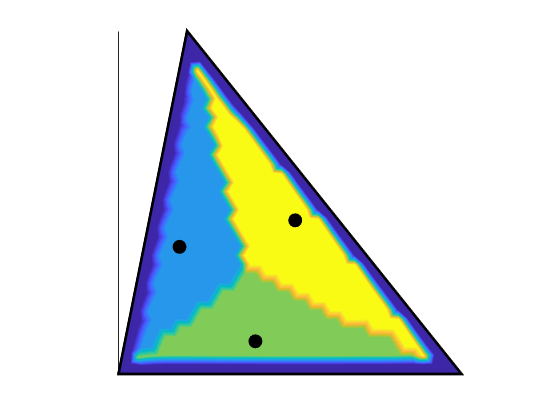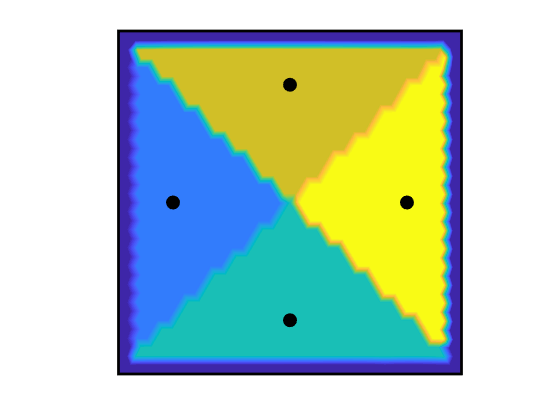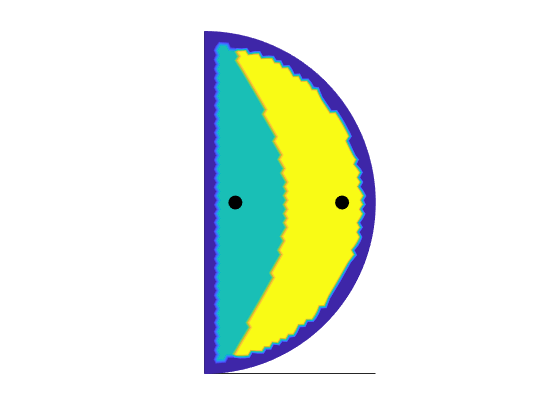# InFocus

## InFocus: A fast inertial lift calculator in MATLAB

InFocus is an easy-to-use tool that can calculate particle focusing positions in a channel of arbitrary cross section after just a few minutes of computation on a desktop computer. The method used draws upon and sharpens asymptotic theory to replace particles by singularities of known sizes. Read more about the algorithm on The Arxiv.

## Examples

Example: Uniform mesh on a triangle

pv = [0  0;  1  0;  0.2  1];  %Polygon Vertices
Re = 1;  %Reynolds Number
[xp, v] = findMigrationPolyChannel(pv,Re);Example: Take advantage of symmetry by specifying points and using reflections

pv = [-0.5  -0.5; -0.5  0.5; 0.5  0.5;  0.5  -0.5;  -0.5  -0.5];
Re = 1;
fh=@(p) min(0.02+max(0,0.5*drectangle(p, -2, 0, -0.5, 0).^3),0.1);  % custom mesh distance that puts more points in one quadrant
[X,Y] =    meshgrid(-0.5:0.05:0,-0.5:0.05:0);
X = reshape(X,numel(X),1);
Y = reshape(Y,numel(Y),1);
xp = [X,Y]';  %particle testing locations
settings = {'reflectx','reflecty'};  %commands to take advantage of symmetry
[xp,v] = findMigrationPolyChannel(pv, Re, fh, xp, settings)Example (Define a channel with curved edges)

fd=@(p) ddiff(dcircle(p,0,0,1),drectangle(p,-1,0,-1,1));
bbox = [0,-1;1,1];
Re = 1
fh=@(p) min(0.02+max(0,0.5*drectangle(p, 0, 1, 0, 1).^3),0.05);
pfix = [0,-1;0,1];
[X,Y] =  meshgrid(0:0.1:1);
X = reshape(X,numel(X),1);
Y = reshape(Y,numel(Y),1);
xp = [X,Y]';
settings = {'reflectx'};
[xp,v] = findMigrationCurveChannel(fd,bbox,pfix, Re,fh,xp,settings)For more instruction on how to construct a boundary function for your channel geometry see DistMesh.